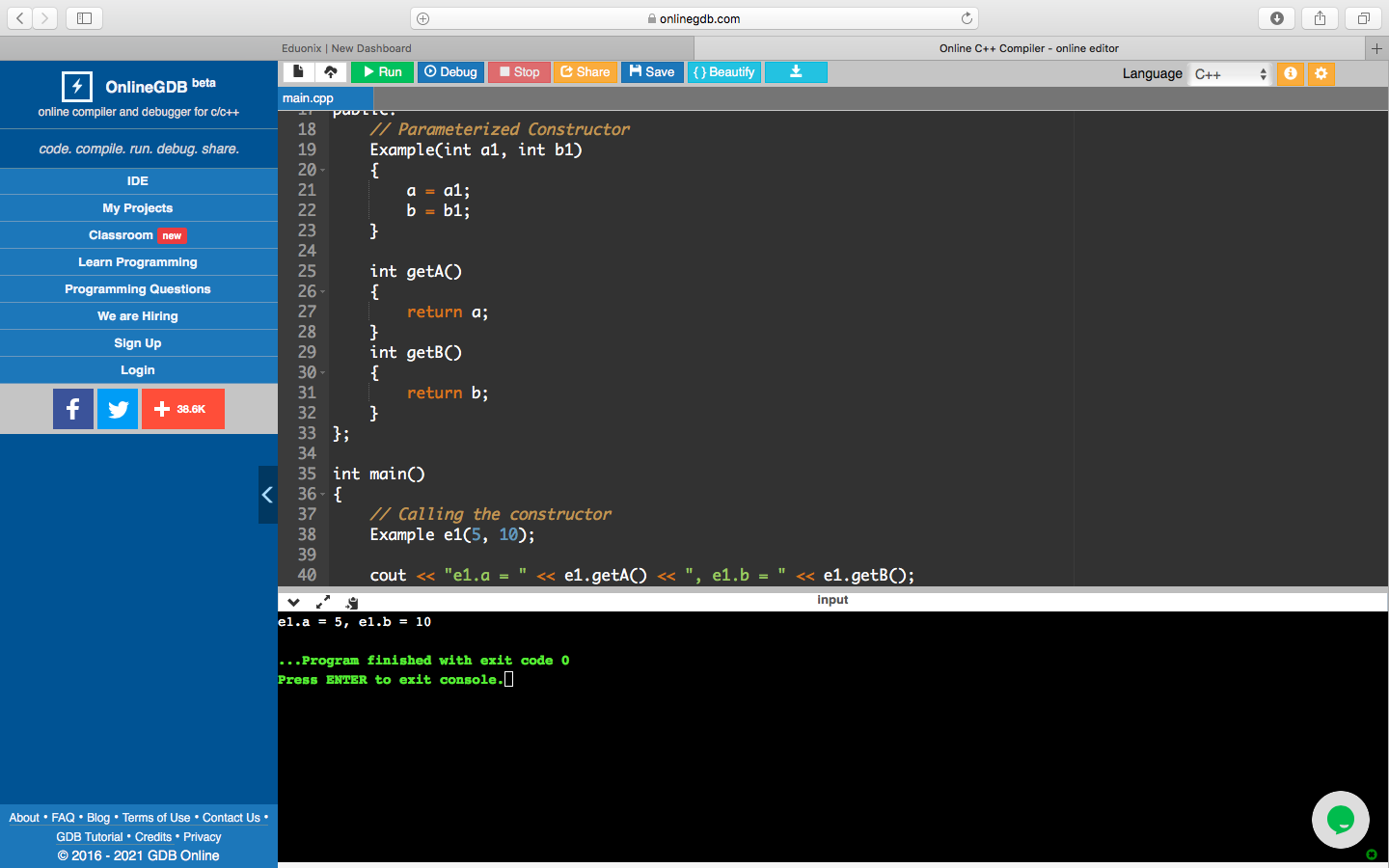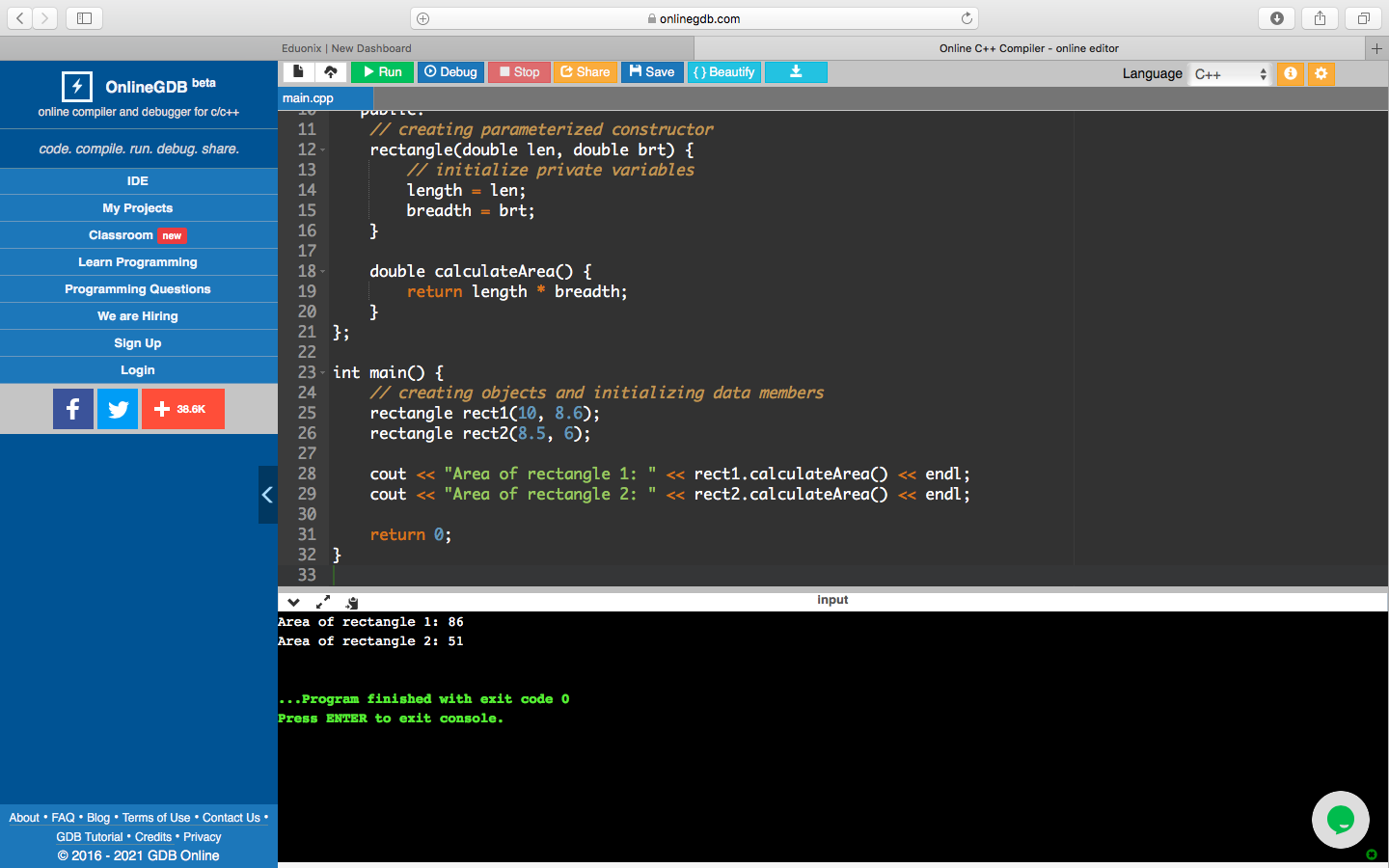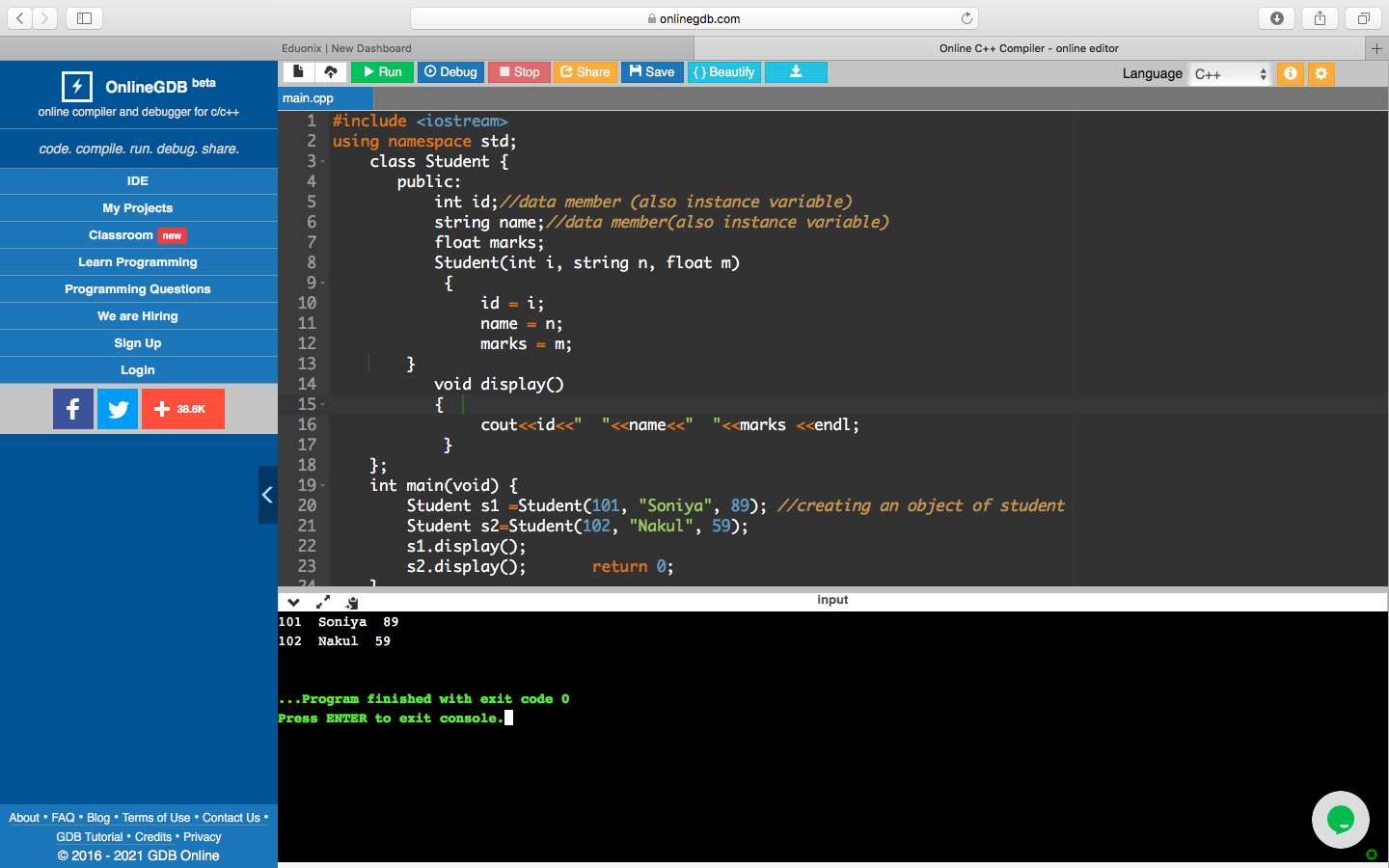# Parameterized Constructor In C++: Working & Examples

## What is Constructor?

A constructor is a class’s member function that is used to initialize objects in a class. In C++, when an object which is the class’s instance, is created, the constructor is called automatically. Thus, a constructor is a special member function of the class.

## What is a Parameterized Constructor?

Arguments can be passed to constructors. When an object is created, these arguments help initialize an object. To create a parameterized constructor in C++, we can add parameters to a function like it can be added to any other function. When the body of the constructor is defined, the parameters are used to initialize the object.

### Syntax of Parameterized Constructor in C++

class name_of_class{

Access specifier (Public/protected/private):

Member variables

Member functions

public:

name_of_class(variables){ //Code for constructor

}

// other functions and variables

}

The syntax included having name_of_class, followed by an access specifier that contains member functions and member variables. All these are included in the constructor code, which means that it can be called in the constructor’s body.

Also Read:  Open Source Projects for C++

### Example of Parameterized Constructor in C++

#include <iostream>

using namespace std;

class Example

{

private:

int a, b;

public:

// Parameterized Constructor

Example(int a1, int b1)

{

a = a1;

b = b1;

}

int getA()

{

return a;

}

int getB()

{

return b;

}

};

int main()

{

// Calling the constructor

Example e1(5, 10);

cout << “e1.a = ” << e1.getA() << “, e1.b = ” << e1.getB();

return 0;

}

OutputExplanation: Private variables a and b are declared in the class Example. A parameterized constructor is declared using the function Example. It includes two methods getA() and getB(). In the main class, the constructor is called, and the constructor’s access values are assigned.

## How Does the Parameterized Constructor in C++ Work?

An object gets initiated, which holds values or the details and parameters the object will process or contain whenever a parameterized constructor in C++ is defined. Then it becomes possible for arguments to be passed to that object. The procedure is very similar to passing a value to a function. It also holds a similarity to passing parameterized values to the objects.

The objects which are defined in the body of the constructor are initialized using the parameters. The values should be passed as arguments to the constructor function whenever a parameterized constructor is declared. These constructors can be called both explicitly or implicitly. The conventional way of object declaration does not work.

Note: Types of call-

1. Explicit call- Example e1= Example(0,10)
2. Implicit call- Example e1(0,10)

Checkout: Project Ideas in C++ for Beginners

## What is the Use of Parameterized Constructor in C++?

The uses of parameterized constructors are as follows:

• Used to assign different values to the various data elements of different objects when they are initialized/created

Another example:

1. Program to calculate the area of a rectangle

#include <iostream>

using namespace std;

// declaring a class

class rectangle {

private:

double length;

public:

// creating parameterized constructor

rectangle(double len, double brt) {

// initialize private variables

length = len;

}

double calculateArea() {

}

};

int main() {

// creating objects and initializing data members

rectangle rect1(10, 8.6);

rectangle rect2(8.5, 6);

cout << “Area of rectangle 1: ” << rect1.calculateArea() << endl;

cout << “Area of rectangle 2: ” << rect2.calculateArea() << endl;

return 0;

}

OutputExplanation: In this example, we have created a parameterized constructor rectangle() with two parameters: double len and double bdt. These parameters contain values that are used to initialize the member variables length and breadth. When we create an object of the Rectangle class, we pass the member variables’ values as arguments. We can calculate the area with the calculateArea() function when the member variables are initialized.

1. Displaying marks of students

#include <iostream>

using namespace std;

class Student {

public:

int std_id;//data member

string std_name;//also instance variable

float std_marks;

Student(int i, string a, float b)

std_id = i;

std_name = a;

std_marks = b;

void display()

cout<<std_id<<”  “<<std_name:<<”  “<<std_marks <<endl;

};

int main(void) {

Student s1 =Student(101, “Soniya”, 89); //creating an object of student

Student s2=Student(102, “Nakul”, 59);

s1.display();

s2.display();         return 0;

}

OutputExplanation: id, name, and marks are data members (also instance variables). A student is a parameterized constructor, and its objects are created in the main class.

## Conclusion

Constructors are just special kinds of methods in which we can pass values. If we do not pass values to the constructor, then the end object has some default value.  [MOU2] The objects hold the methods and values of member variables that are part of the main class. These values are finally passed via constructors. A parameterized constructor in C++ has its advantage of assigning different objects different values, and there can be overloading.

Now that you are aware of the parameterized constructors in C++, if you want to dig deeper and move up in your programming career, then explore courses from upGrad, India’s largest online higher education company. You must check our Full-Stack Software Development Programme.

## Prepare for a Career of the Future

UPGRAD AND IIIT-BANGALORE'S FULL STACK SOFTWARE DEVELOPMENT PROGRAMME
APPLY NOW

## 0 replies on “Parameterized Constructor In C++: Working & Examples”